Write the number of neutrons present in C and C isotopes of carbon

1. Given that natural sample of iron has isotopes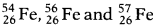in the ratio 5%, 90% and 5% respectively. What will be the average atomic mass of Fe? Write any two applications of isotopes.
• The relative atomic mass of boron is 10.8. Calculate the percentage of its isotopes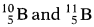occurring in nature.
• Write the number of neutrons present in C and C isotopes of carbon.
1. Average atomic mass of Fe =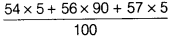=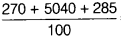=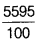= 55.95 u
Two applications of isotopes are
• C-14 is used to determine the age of old samples of fossils.
• Co-60 is used in the treatment of cancer (radiotherpy).
• Let the percentage of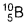be Y in the sample and the percentage of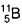will be (100 - Y) .
Average mass =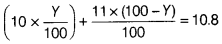10Y + 11(100 - Y) = 1.08 x 100
10Y + 1100 - 11Y = 108
⇒ Y = 20
Therefore, the percentage of= 20% and percentage of= 100 - 20 = 80%.
• Number of neutron in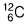= Mass number - atomic number = 12 - 6
Number of neutrons in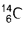= 14 - 6 = 8